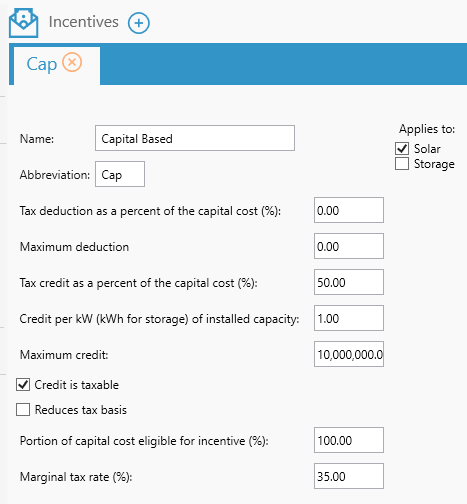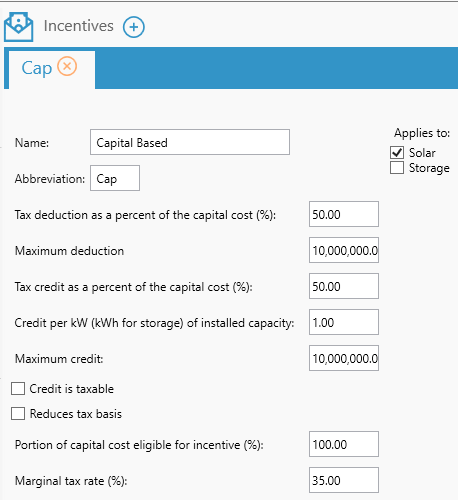# HOMER Grid 1.1

 Navigation: Design > Incentives Capital BasedCapital based incentives apply to the capital cost of the components. This could either be a tax deduction or a credit or both.

In HOMER Grid, you can apply a capital based incentive to the following components:

oSolar

oStorage

Below is an explanation of the various inputs in capital based incentive

 Variable Description Percent deduction (%) Tax deduction as a percent of the capital cost Maximum deduction Maximum deduction limit Percent credit (%) Tax credit as a percent of the capital cost Credit per kW (per kWh for storage) Additional credit per kW/kWh of installed capacity Maximum credit Maximum credit allowed Eligible percent (%) Portion of capital cost eligible for incentive Marginal tax percent (%) The percent of tax applicable Credit is taxable Select this option if the credit is reduced by the marginal tax rate Reduces tax basis Select this option if deduction and credit reduces the tax basis (cost basis) Applies to The components that this bonus depreciation applies to

Below are two examples of capital based incentives:

## Example 1

Let us consider a PV system of 100 kW which has a total capital cost of 100,000\$. If the capital based incentive applied to this PV system is:This would mean that the tax credit applied to the system is = 50% of capital cost = 0.50 * 100,000\$ = 50,000\$

Additional credit per kW of installed capacity = 1 \$ * 100 kW (installed capacity) = 100 \$

Total credit = 50,000\$ + 100\$ = 50,100\$

Since the "Credit is taxable"  is selected, the marginal tax rate is applied to this credit = (1 - 0.35) * 50,100 \$ = 32,565 \$

Total Capital based incentive = 32,565 \$

## Example 2:

Let us consider a PV system of 100 kW which has a total capital cost of 100,000\$. If the capital based incentive applied to this PV system is:This would mean that the tax credit applied to the system is = 50% of capital cost = 0.50 * 100,000\$ = 50,000\$

Additional credit per kW of installed capacity = 1 \$ * 100 kW (installed capacity) = 100 \$

Total credit = 50,000\$ + 100\$ = 50,100\$

Since the Tax deduction is 50 % of capital cost = 0.50 * marginal tax rate * capital cost = 0.50 * 0.35 * 100,000\$ = 17,500\$

Total deduction and credit = 17,500 + 50,100 \$ = 67,600 \$

The Homer Support site has a searchable knowledgebase and additional support options. HOMER Online contains the latest information on model updates, as well as sample files, resource data, and contact information. ©2017 HOMER Energy, LLC